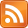SMS scnews item created by Daniel Daners at Sat 7 Mar 2020 1137
Type: Seminar
Distribution: World
Expiry: 9 Mar 2020
Calendar1: 9 Mar 2020 1400-1500
CalLoc1: AGR Carslaw 829
CalTitle1: PDE Seminar: Coupled systems of heat equations and convergence to equilibrium (Glueck)
Auth: daners@d27-99-2-95.bla1.nsw.optusnet.com.au (ddan2237) in SMS-SAML

# Coupled systems of heat equations and convergence to equilibrium

### Glueck

Jochen Glück
University of Passau, Germany
Mon 9th Mar 2020, 2-3pm, Carslaw Room 829 (AGR)

## Abstract

On a bounded domain in $\Omega \subseteq {ℝ}^{d}$, consider the coupled heat equation

subject to Neumann boundary conditions, where $V:\Omega \to {ℝ}^{N×N}$ is a matrix-valued potential. While the solution to a single heat equation is well-known to converge to an equilibrium as $t\to \infty$, the matrix potential $V$ can for instance introduce the existence of periodic solutions to the equation.

In this talk, we will discuss sufficient conditions for the solutions to the above equation to converge as $t\to \infty$. We shall see that well-behavedness of the potential $V$ with respect to the ${\ell }^{p}$-unit ball in ${ℝ}^{n}$ is a crucial property, here – more precisely speaking, we need that $V$ is $p$-dissipative.

What makes our analysis quite interesting is the fact that we need completely different methods for the cases $p=2$ and $p\ne 2$: in the first case, standard Hilbert space techniques can be used, while the case $p\ne 2$ requires more sophisticated methods from spectral geometry, the geometry of Banach spaces and semigroup theory.

This talk is based on joint work the Alexander Dobrick (Christian-Albrechts-Universität zu Kiel)

For Seminar announcements you can now subscribe to theSeminar RSS feed. Check also the PDE Seminar page.

Enquiries to Daniel Hauer or Daniel Daners.